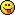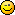###Author Topic: Trying to extend GLB types(mainly C++)  (Read 2816 times)

####bigsofty

• Community Developer
• Prof. Inline
••• Posts: 2681##### Trying to extend GLB types(mainly C++)
« on: 2012-Jul-31 »
Edit: *** Experimental, don't use in your own code ***

Started as messing about but it would be nice if it could work as it would be great to have operator overloading with types.

Consider this type:

Code: (glbasic) [Select]
`TYPE TVec2  x# y# w# = 1ENDTYPE`
Which when compiled by GLB creates this:

Code: (glbasic) [Select]
`class TVec2{public: DGInt x; DGInt y; DGInt w; TVec2() { x= 0; y= 0; w= 1; } TVec2(const TVec2& _in_) {*this = _in_;} TVec2& operator=(const TVec2& _in_) { this->x = _in_.x; this->y = _in_.y; this->w = _in_.w; return *this; } bool operator <(const TVec2& _in_)const { if(this->x < _in_.x) return true; return false; } bool operator >(const TVec2& _in_)const { if(this->x > _in_.x) return true; return false; } bool operator==(const TVec2& _in_)const { return (1 && x == _in_.x && y == _in_.y && w == _in_.w )?true:false; } bool operator!=(const TVec2& _in_)const { return !(*this==_in_); }};`

So I wondered if I could add a "+" operator overload function but to I soon found out that any inline within my type was not added to my TVec2 class, which was a pity, it was stuck outside a class with the globals and other code.

So I tried to create and external class operator overload function, something like this...

Code: (glbasic) [Select]
`TYPE TVec2  x# y# w# = 1INLINE  TVec2 operator+(TVec2 _a, TVec2 const& _b ) { _a.x += _b.x; _a.y += _b.y; _a.w += _b.w; return _a; }ENDINLINEENDTYPE`
Which compiles but the GLB.H file has a type check for valid types that kills off this...

Code: (glbasic) [Select]
` LOCAL a AS TVec2 LOCAL b AS TVec2 a=a+b`
At this point my limited C++ knowledge is letting me down.

Any ideas?
« Last Edit: 2012-Aug-02 by bigsofty »
Cheers,

Ian.

“It is practically impossible to teach good programming style to students that have had prior exposure to BASIC.  As potential programmers, they are mentally mutilated beyond hope of regeneration.”
(E. W. Dijkstra)

####Quentin

• Prof. Inline
•• Posts: 915##### Re: Trying to extend GLB types(mainly C++)
« Reply #1 on: 2012-Jul-31 »
it could work, if you pay attention to some issuesplace the +operator outside the type as a normal C++ function
in this case a forward declaration is needed (see first INLINE statement)

it's also possible to define different +operators (e.g. type + type or type + number)

Code: (glbasic) [Select]
`INLINEconst TVec2 operator+(TVec2& _a, const TVec2& _b );const TVec2 operator+(TVec2& _a, DGInt b);ENDINLINETYPE TVec2 x# y# w# = 1ENDTYPELOCAL one AS TVec2LOCAL two AS TVec2one.x = 1one.y = 2two.x = 3two.y = 4LOCAL result AS TVec2result = one + tworesult = result + 11STDOUT "result.x = " + result.x + "\n"STDOUT "result.y = " + result.y + "\n"STDOUT "result.w = " + result.w + "\n"KEYWAITFUNCTION __dummy__:ENDFUNCTIONINLINE  const TVec2 operator+(TVec2& _a, const TVec2& _b ) { _a.x += _b.x; _a.y += _b.y; _a.w += _b.w; return _a; } const TVec2 operator+(TVec2& _a, DGInt b) { _a.x += b; _a.y += b; _a.w += b; return _a; }ENDINLINE`
[EDIT]
The usage of const is quite confusing for me. In many examples I see both parameters definded as const. Not really sure, which is the best way. But I guess in this case it's ok to use _a without const to avoid a temporay copy of TVec2.
« Last Edit: 2012-Jul-31 by Quentin »

####Albert

• Dr. Type
••• Posts: 257##### Re: Trying to extend GLB types(mainly C++)
« Reply #2 on: 2012-Jul-31 »
Maybe we can add some sort of inheritence too?

• Guest##### Re: Trying to extend GLB types(mainly C++)
« Reply #3 on: 2012-Jul-31 »
Gernot doesn't like the idea####bigsofty

• Community Developer
• Prof. Inline
••• Posts: 2681##### Re: Trying to extend GLB types(mainly C++)
« Reply #4 on: 2012-Jul-31 »
Well done Quentin, I never thought of using const, I was trying a template but if this works then it opens up vector and matrix types to GLB.

I,ll have a go later tonight, when I'm near my PC.
Cheers,

Ian.

“It is practically impossible to teach good programming style to students that have had prior exposure to BASIC.  As potential programmers, they are mentally mutilated beyond hope of regeneration.”
(E. W. Dijkstra)

####bigsofty

• Community Developer
• Prof. Inline
••• Posts: 2681##### Re: Trying to extend GLB types(mainly C++)
« Reply #5 on: 2012-Aug-01 »
OK, a little bit more, the only change I had to do was change the first param from a reference to a value. (This allows GLB to parse more then one operator per vector calculation, more than one(result=one*two/three) would produce a running value, wich the next parsed operator would then use.)

So longer vector calculations are now possible(see "result = (((one + two) * two) * 10) / 4 + one", try this with the previous method to see the change)

Still messing around with this, next problem is overloading Gernots auto-generated operators as shown above >,<,=,==,!= etc.

Code: (glbasic) [Select]
`// --------------------------------- //// Project: veclibTest// Start: Tuesday, July 31, 2012// IDE Version: 11.001INLINE const TVec2 operator+(TVec2 _a, const TVec2& _b ); const TVec2 operator+(TVec2 _a, DGInt b); const TVec2 operator*(TVec2 _a, const TVec2& _b ); const TVec2 operator*(TVec2 _a, DGInt b); const TVec2 operator/(TVec2 _a, const TVec2& _b ); const TVec2 operator/(TVec2 _a, DGInt b);ENDINLINETYPE TVec2 x# y# w# = 1ENDTYPELOCAL one AS TVec2LOCAL two AS TVec2one.x = 1one.y = 2two.x = 3two.y = 4LOCAL result AS TVec2result = (((one + two) * two) * 10) / 4 + oneSTDOUT "result.x = " + result.x + "\n"STDOUT "result.y = " + result.y + "\n"STDOUT "result.w = " + result.w + "\n"KEYWAITFUNCTION __dummy__:ENDFUNCTIONINLINE  const TVec2 operator+(TVec2 _a, const TVec2& _b ) { _a.x += _b.x; _a.y += _b.y; return _a; } const TVec2 operator+(TVec2 _a, DGInt b) { _a.x += b; _a.y += b; return _a; }  const TVec2 operator*(TVec2 _a, const TVec2& _b ) { _a.x *= _b.x; _a.y *= _b.y; return _a; } const TVec2 operator*(TVec2 _a, DGInt b) { _a.x *= b; _a.y *= b; return _a; }   const TVec2 operator/(TVec2 _a, const TVec2& _b) { _a.x /= _b.x; _a.y /= _b.y; return _a; } const TVec2 operator/(TVec2 _a, DGInt b) { _a.x /= b; _a.y /= b; return _a; }ENDINLINE`
Cheers,

Ian.

“It is practically impossible to teach good programming style to students that have had prior exposure to BASIC.  As potential programmers, they are mentally mutilated beyond hope of regeneration.”
(E. W. Dijkstra)

####Kitty Hello

• code monkey
• Prof. Inline
••• Posts: 10746
• here on my island the sea says 'hello'##### Trying to extend GLB types(mainly C++)
« Reply #6 on: 2012-Aug-02 »
Will the precompiler allow you to use these operators on types? That's an uncatched error, because w/o your inline it will return ab gcc error.

I know operators are nicer to use but it's no longer BASIC, I think.

####Minion

• Mr. Polyvector
•• Posts: 229##### Re: Trying to extend GLB types(mainly C++)
« Reply #7 on: 2012-Aug-02 »
...
I know operators are nicer to use but it's no longer BASIC, I think.

Nuff said

####bigsofty

• Community Developer
• Prof. Inline
••• Posts: 2681##### Re: Trying to extend GLB types(mainly C++)
« Reply #8 on: 2012-Aug-02 »
Will the precompiler allow you to use these operators on types? That's an uncatched error, because w/o your inline it will return ab gcc error.

I know operators are nicer to use but it's no longer BASIC, I think.

Well this is just an experiment, as I've now noted in thread one.

Actually a lot of the more recent Basics support operator overloading (Freebasic, Visual Basic, Monkey for example).

I admit the example that is used above is gibberish, made to test the type manipulation its working right, rather than have any functional sense(Also the variable names are terrible!)

But it DOES incredibly improve the handling of more complex types and makes the resultant code a breeze to use and understand(the object code produced is very efficient too).

Functional overloading is not supported in GLBasic. In this case Scalars and Vectors are mixed freely and invisibly, this is not possible in GLBasic without separate functions dependant on their types. This becomes a real problem when mixing the sub-results for more complex formula.

Simplicity of syntax, well I'll use the above, gibberish example(with really bad variable names!) as an example of the difference in doing this with nothing but functions. Here is a new line based on the above. I would added the "add/sbt/mul/div" to a type but this would have mad the formula even more unreadable with the extra type declaration.

Code: (glbasic) [Select]
`// Overloadedresult = (((one + two) * two) * 10) / 4 + one// Currentresult = AddVV(DivVS(MulVS(MulVV(AddVV(one,two),two),10),4),one) //VV=vec to vec, VS=Vec to Scalar`
I guarantee that this would not work, as GLB has a lot of trouble with nested function results.

I ask this not be be 'fixed' as this could easily be a nice 'feature' as opposed to a 'bug' as Microsoft would say!Here is the latest version with a little more functionality towards a decent Vector type...